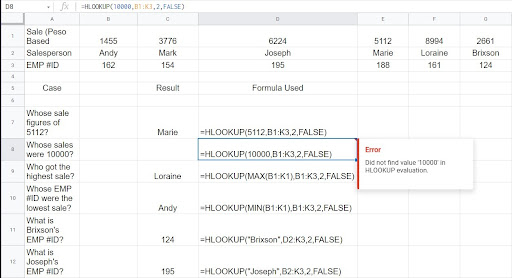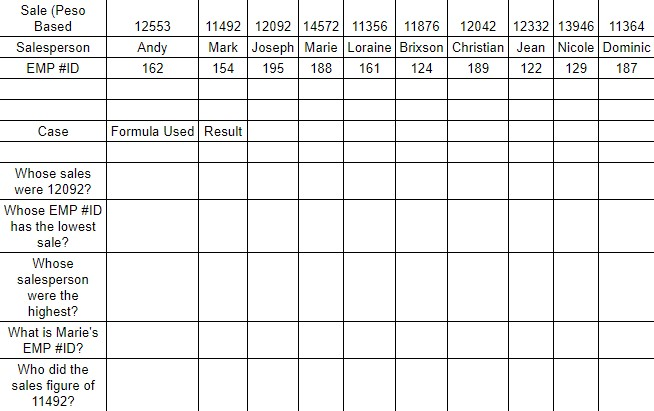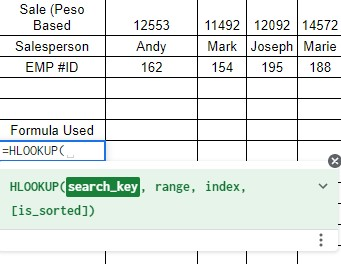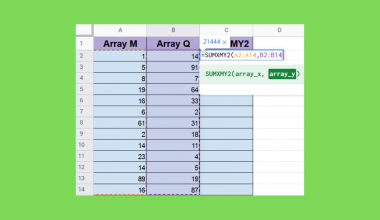# How to use HLOOKUP in Google Sheet

The HLOOKUP function in Google Sheets looks for a critical value in the first row of the input range

It then returns the value of a specific cell from the column where it finds the key. If the key does not exist inside the range, an error will be generated.

The rules for using the `HLOOKUP` function in Google Sheet are as follows:

• Ensure that the first row in the range where you want to extract the values (e.g., numeric or date values) is not written as texts. Always check its format. Otherwise, the `HLOOKUP` function will give you an error message or an erroneous return value.
• The `HLOOKUP` function does not support regular expressions and wildcard patterns-based search keys.

Let’s check this example below.Marie was tasked to analyze data about their sales, employee ID, and employees’ name from the google sheet. However, the google sheet spreadsheet has a wide range and multiple values on it.

Marie then utilizes the `HLOOKUP` function to filter and narrow down the range. Within seconds, Marie gets all of the data that she needs.

I will teach you the trick Marie used to quickly pull up the information. But first, let us learn the basics.

## The Anatomy of the HLOOKUP Function in Google Sheets

So, the syntax (the way we write) of the `HLOOKUP` function is as follows:

=HLOOKUP(search_key, range, index [is sorted])

Let’s dissect its parts and understand how the function works.

• = every function in the google sheet always starts with an equal sign.
• Search key — the value that the `HLOOKUP` function searches for.
• Range – refers to the range of cells on which we are searching.
• Index – the row index of the cell inside the range whose value is returned by the function. The index of the first row inside the range is one, the index of the second row within the range is two, and so on.
• [is sorted] – is an optional argument with a default value “TRUE.”

## A REAL Example of Using HLOOKUP Function in Google Sheets

There are a couple of examples of how to use the `HLOOKUP` function.

This article will use the most common scenario where the `HLOOKUP` function is utilized.

Number in the first row:A test data set including sales figures for a group of salespeople may be found here. We will use `HLOOKUP` function to answer a variety of business case issues. We will see how the value of the index parameter influences the result.

The function is told to look for the value 5112 inside the range of cells B1 to K3 using the formula =`HLOOKUP`(5112, B1:K3,2, False). When it discovers the value, it is told to put it in the second row of the column where it was found. False means the data is not sorted, and we are looking for an exact match to the search key.

In the last case (row 11 above), there is something worth noting. The function returned an error code of #N/A. Why? The error explanation indicates that the \$10000 sales amount does not exist in the first row. It cannot provide an adequate response because we want an exact match (using False for the sorted option).

Now we will have a quick practice. Click on the link below and copy the sample spreadsheet.

Let us now practice using the `HLOOKUP` function.

## How to use HLOOKUP Function in Google Sheets

1. Before you input the `HLOOKUP` function, make sure that all data in the source spreadsheet is correct.

In this example, we use this data:1. Now, when we input the `HLOOKUP` function, we must start it with an ‘‘sign. Otherwise, the Google Sheet spreadsheet will not know that we are working on a specific function and not a text.1. After putting the first part of the function, we will add the Search key, which will trigger Google Sheets to look up the value we are searching for.1. Once we key in the Search key, we will now input the range of the cells where we want to search.1. Once done, we will add this in the function [is sorted]. Depending on the value that we want to achieve, we can either use TRUE or FALSE. This function specifies whether the range’s first row is sorted in ascending or descending order.1. To complete the `HLOOKUP` function and inform google sheet what we specifically want it to look up, add a close ‘)‘parenthesis at the end of the function.What is the difference between `HLOOKUP` function and `VLOOKUP` function?

The `HLOOKUP` function is not as well known or commonly used as its counterpart, the `VLOOKUP` function.  However, the two functions are identical, with the `HLOOKUP` function merely being a transposed version of `VLOOKUP`

`HLOOKUP` stands for ‘Horizontal Lookup.’

The biggest distinction from `VLOOKUP` to `HLOOKUP` would be that `VLOOKUP` is used when the table is set up vertically, whereas `HLOOKUP` is used when the table is set up horizontally.

That’s it! That’s another Google Sheet spreadsheet trick for you. You may also check this helpful link to know more about the `VLOOKUP` function in Google Sheet.Our goal this year is to create lots of rich, bite-sized tutorials for Google Sheets users like you. If you liked this one, you'll love what we are working on! Readers receive ✨ early access ✨ to new content.

##### You May Also Like## How to Insert Excel Table in Gmail with Borders

Occasionally you’ll want to insert an Excel table directly into your Gmail. Besides the fact that it looks…## How to Use UPPER Function in Google Sheets

The UPPER function in Google Sheets is useful if you want to convert a given text to all…## How to Use the MIRR Function in Google Sheets

The MIRR function in Google Sheets is used to calculate the modified internal rate of return on an…## How to Use SUMIFS Function in Google Sheets

The SUMIFS function in Google Sheets is useful if you want to get the sum of cells that…